## research papersBIOLOGICALCRYSTALLOGRAPHY
ISSN: 1399-0047
Volume 62| Part 1| January 2006| Pages 48-57

## The integration of macromolecular diffraction data

aMRC Laboratory of Molecular Biology, Hills Road, Cambridge CB2 2QH, England
*Correspondence e-mail: andrew@mrc-lmb.cam.ac.uk

(Received 19 May 2005; accepted 24 November 2005)

The objective of any modern data-processing program is to produce from a set of diffraction images a set of indices (hkls) with their associated intensities (and estimates of their uncertainties), together with an accurate estimate of the crystal unit-cell parameters. This procedure should not only be reliable, but should involve an absolute minimum of user intervention. The process can be conveniently divided into three stages. The first (autoindexing) determines the unit-cell parameters and the orientation of the crystal. The unit-cell parameters may indicate the likely Laue group of the crystal. The second step is to refine the initial estimate of the unit-cell parameters and also the crystal mosaicity using a procedure known as post-refinement. The third step is to integrate the images, which consists of predicting the positions of the Bragg reflections on each image and obtaining an estimate of the intensity of each reflection and its uncertainty. This is carried out while simultaneously refining various detector and crystal parameters. Basic features of the algorithms employed for each of these three separate steps are described, principally with reference to the program MOSFLM.

### 1. Introduction

The collection of macromolecular diffraction data has undergone dramatic advances during the last 15 years with the advent of two-dimensional area detectors such as image plates and CCDs, crystal cryocooling and the availability of intense, monochromatic and highly collimated X-ray beams from synchrotron sources. These technical developments have been accompanied by significant advances in the software used to process the resulting diffraction images. In particular, autoindexing procedures have improved the ease of data processing to the point that in many cases it can be carried out automatically without any user intervention. However, the procedure used to collect the diffraction images, the screenless rotation method, has remained essentially unchanged since it was first suggested for macromolecular crystals by Xuong et al. (1968) and by Arndt and coworkers and popularized by the availability of the Arndt–Wonacott oscillation camera (Arndt et al., 1973; Arndt & Wonacott, 1977). In this procedure, each diffraction image is collected while rotating the crystal by a small angle (typically between 0.2 and 2°) about a fixed axis (often referred to as the φ axis). The only development of the method has been the use of very small rotation angles per image (the so-called fine φ-slicing technique) to provide improved signal to noise for weakly diffracting samples. Since virtually all macromolecular diffraction data are collected in this way (with the exception of data collected using the Laue technique), this paper will be restricted to the fundamentals of processing images collected using this approach, commonly known as the rotation or oscillation method (the terms are used interchangeably).

The starting point for data integration will therefore be a series of such diffraction images and the desired outcome is a data set consisting of the Miller indices (hkl) of all reflections recorded on these images together with an estimate of the diffracted intensities I(hkl) and their standard uncertainties σI(hkl). This requires the prediction of which reflections occur on each image and also the precise position of each reflection on each image (note that typically most reflections will be present on several adjacent images and therefore only partially recorded on any individual image; see Fig. 1). For each predicted reflection, the background-subtracted diffracted intensity must be estimated. Although straightforward in principle, defects and limitations in both the sample (the crystal) and the detector can make this difficult in practice. Complicating factors include crystal splitting, anisotropic and/or very weak diffraction, high mosaicity, diffuse scattering, the presence of ice rings or spots, unresolved or overloaded spots, noise arising from cosmic rays or zingers, backstop shadows, detector blemishes, radiation damage and spatial distortion. Although these experimental factors will be important in determining the final quality of a data set, they will not be discussed here.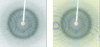Figure 1 A typical macromolecular diffraction pattern for a strongly diffracting crystal. The original image is shown on the left, with the predicted reflections shown superposed on the right. Each reflection is shown as a box, colour-coded blue and yellow for fully recorded and partially recorded reflections, respectively, and green for reflections with a width greater than 5°.

It is convenient to subdivide the process of integrating the diffraction images into three stages. The first is the determination of the crystal parameters, in particular the crystal lattice (unit cell) and its orientation relative to a laboratory axial system (usually based on the X-ray beam direction and the rotation axis). This is usually referred to as autoindexing. Knowledge of these parameters then allows an initial estimate of the crystal mosaicity. The second step is the determination of accurate unit-cell parameters, using a procedure known as post-refinement. This requires the integration of one or more segments of data with a few images in each segment. The final step is the integration of the entire set of diffraction images, while simultaneously refining parameters associated with both the crystal and the detector. The underlying principles of these three steps are described below.

### 2. Autoindexing

The introduction of autoindexing was one of the most significant advances in simplifying the task of data processing. Prior to this, the unit cell was normally determined by precession methods and the crystal orientation was determined from a series of still (zero oscillation angle) images recorded from a crystal whose approximate orientation was known from the crystal morphology. This process was both laborious and time-consuming.

A number of different autoindexing procedures have been described (Kim, 1989; Higashi, 1990; Kabsch, 1993), but the discussion here will be restricted to the method based on one-dimensional fast Fourier transforms and originally implemented in the DPS program package (Steller et al., 1997), but which is now used in MOSFLM (Leslie, 1992) and d*TREK (Pflugrath, 1999). A similar method but using a three-dimensional Fourier transform is implemented in DENZO and HKL2000 (Otwinowski & Minor, 2001). The spot positions in an oscillation image are a distorted projection of the reciprocal lattice of the crystal. Using the Ewald sphere construction (Fig. 2), it is straightforward to derive the scattering vector (reciprocal-lattice vector) of a reflection from the measured spot coordinates (Xd, Yd) relative to the direct-beam position,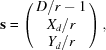where r = (Xd2 + Yd2 + D2)1/2 and D is the crystal-to-detector distance. Note that s is in dimensionless reciprocal-lattice units and the corresponding Ewald sphere radius is unity.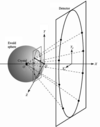Figure 2 The Ewald sphere construction. The X-ray beam is along the X axis and the Z axis is the rotation axis. The origin of the reciprocal lattice lies at the point that the X-ray beam exits the Ewald sphere (Q) and the crystal is located at the centre of the sphere (O). The crystal is oriented so that the a axis lies along the X axis. The reciprocal-lattice plane h = 1 is shown. Each diffraction spot on the detector can be mapped back to the equivalent scattering vector in reciprocal space. One such scattering vector is shown as a bold line from the reciprocal-lattice origin (Q) to the surface of the Ewald sphere.

This equation holds when the centre of the reciprocal-lattice point (which has a finite size associated with the finite reflecting range; see §4.2) lies exactly on the Ewald sphere. Different reflections will have different φ values and so it is necessary to place all the scattering vectors in a common reference frame by correcting for this.

However, when using a single image, there is no reliable way of knowing the φ values for different reflections. For example, if the oscillation image was recorded with a starting φ of 0.0° and an final φ of 1.0°, the true φ value for any individual reflection could lie anywhere between −/2 and 1.0 + /2, where is the reflection width in φ (which will be different for different reflections). Therefore, in practice the φ values for all reflections on a given image are assigned to the midpoint of the rotation for that image (0.5° in the example above). This will introduce small errors into the derived scattering vectors. If more than one image is being used in the autoindexing, then the correction for the φ values of the different images is clearly important.

The general principle behind Fourier-based autoindexing can be understood as follows. Fig. 2shows the Ewald sphere construction for a crystal oriented so that a principal zone axis, in this case the a axis, lies along the X-ray beam direction. The planes of reciprocal-lattice points (h = 1, h = 2, h = 3 etc.) normal to this zone axis intersect the Ewald sphere in a series of concentric circles centred on the direct-beam position. In the diffraction image, a series of concentric lunes will be seen. As described above, all the spots on the detector can be mapped back to give the corresponding scattering vectors. When these scattering vectors are projected onto the zone axis, all the spots lying within the same lune will give rise to a projected vector of the same length. Thus, the projected scattering vectors for all the spots on the image will fall into clusters, where the separation between each cluster corresponds to the vector between adjacent reciprocal-lattice planes (h = 1, h = 2, h = 3 etc.). Because of the regularity of these clusters, the Fourier transform of the projected scattering vectors will form a series of regularly spaced large maxima (Fig. 3), where the distance between adjacent maxima corresponds to the real cell spacing along the principal zone axis direction (the a axis in this example). If, however, the scattering vectors are projected along a direction at an angle of (say) 10° to the true zone axis direction, spots in the same lune will project to give vectors of different lengths, so the Fourier transform of the projected scattering vectors will not have a clear set of maxima. Thus, the height of the maxima in the Fourier transform can be used to identify directions that correspond to real-space zone axes, such as the ab or c axes of the unit cell or low-order vectors such as a + b, a + c, b + c etc. Although for ease of representation it was assumed in this example that the zone axis lies along the X-ray beam direction, this is not a requirement. In addition, an oscillation image can be used for the indexing rather than a still (zero oscillation angle) image, even though this will lead to larger errors in the derived scattering vectors owing to the assumption that all reflections have the same φ value.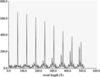Figure 3 A one-dimensional Fourier transform of projected scattering vectors. With the crystal orientation as shown in Fig. 2, the scattering vectors projected onto the X axis will consist of regularly spaced clusters corresponding to reciprocal-lattice planes h = 1, h = 2, h = 3 etc. The Fourier transform will consist of several large discrete maxima, where the spacing between adjacent maxima corresponds to the real cell spacing, in this case the a axis.

In practice, the direction of the projection axis is varied in small angular steps (e.g. 2°) for the complete hemisphere of directions centred on the X-ray beam and in each case the Fourier transform of the projected scattering vectors is calculated. Three directions are then chosen from this list that have large maxima in the Fourier transform and reasonably large inter-axial angles and the directions optimized by a fine angular search. These will define three principal zone axes and their repeats, thus defining a unit cell with which it should be possible to index all spots in the diffraction image. In general, the resulting unit cell will be a triclinic one that will not reflect the true symmetry of the lattice. The final stage is therefore to find the reduced cell from the chosen cell and then evaluate a goodness of fit (penalty score) to the 44 possible lattice types (Burzlaff et al., 1992). The user is presented with a list of possible solutions, each with a corresponding penalty. Typically, there will be a number of solutions with a low penalty and then further solutions with much higher penalties; usually the solution with the highest Bravais lattice symmetry in the group with low penalty scores will be correct. The unit-cell parameters are then refined (using the observed spot positions) imposing any constraints appropriate for the lattice symmetry of the chosen solution. The direct-beam parameters and (optionally) the crystal-to-detector distance are refined at the same time.

It is important to realise that there is no information available at this stage on the true crystal symmetry, which can only be determined from the diffraction intensities. The spot positions only give information about the lattice symmetry, which can be higher than the true crystal symmetry. This is particularly important when considering the strategy for data collection.

Success of the autoindexing depends critically on knowledge of experimental parameters such as the wavelength of the radiation, the crystal-to-detector distance and most importantly the direct-beam coordinates (however, see Sauter et al., 2004for recent improvements in this respect). Accurate knowledge of the direct-beam position is particularly important when processing data from crystals with one or more large unit-cell edges, as it may not be possible to detect mis-indexing by a single index from the integration statistics (although this will be clear when the data is merged). Ideally a few hundred spots should be used for indexing, although in favourable cases as few as 50 can be sufficient. In cases where two lattices are present, it may be possible to index the stronger lattice simply by applying an intensity cutoff when selecting spots to be used in indexing; otherwise, spots from one of the lattices can be selected manually. Crystals with very high mosaicity can present difficulties if this gives rise to the overlap of spots in adjacent lunes. Because the φ values of individual reflections are not known, this will give rise to serious errors in the derived scattering vectors and a failure of the algorithm. Experience has shown that the indexing is more robust when two images are used (preferably separated by 90° in φ) and results derived from a single image can be misleading in some cases, particularly for low symmetries such as monoclinic. The absence of a clear separation in penalty score between solutions with low penalty and those with higher penalties may indicate that the true cell is triclinic, but can also arise if there are errors in the experimental parameters, such as the direct-beam position.

### 3. Estimating the mosaic spread

The final error in predicted spot positions after cell refinement is a good indicator of the success or failure of the autoindexing, but the definitive test is to compare the predicted pattern of spots with the observed diffraction image. At this stage not all spots will be predicted, because the mosaicity of the crystal is not known (and assumed to be zero), but there should be general agreement in the position of the lunes and the separation of adjacent spots. Assuming that the prediction is correct, the mosaic spread can be estimated by measuring the total intensity of all predicted spots for increasing values of the mosaicity (typically from 0 to 2° in steps of 0.2°). The total intensity should reach a maximum at the correct value of the mosaic spread, as larger values of the mosaic spread will simply predict reflections that are not present. In practice, the total intensity does not reach a constant value at the correct mosaic spread owing to diffuse scatter in the diffraction image, but it does increase much more slowly as the mosaic spread is increased further. This allows a reasonable starting estimate to be determined, which is subsequently refined by post-refinement.

### 4. Parameter refinement

Once an orientation matrix and unit-cell parameters have been derived from the autoindexing, these parameters (and others) are refined further using different algorithms. The parameters to be refined can be conveniently grouped into three classes.

 (i) Crystal parameters: unit-cell parameters, crystal orientation and mosaic spread (isotropic or anisotropic). (ii) Detector parameters: the detector position and orientation and (if appropriate) distortion parameters (e.g. the radial and tangential offsets for the MAR Research image-plate scanner). (iii) Beam parameters: the orientation of the primary beam and beam divergence (isotropic or anisotropic).

There are two complementary sources of information that can be used in the refinement: the spot coordinates measured on the detector and the spot coordinates in φ. The latter can be estimated empirically if the oscillation angle is much smaller than the reflection width or can be estimated from the way in which the intensity for partially recorded reflections is distributed over the two (or more) images on which the reflection is recorded if the oscillation angle is comparable to, or greater than, the reflection width.

#### 4.1. Refinement using spot coordinates measured on the detector

The parameters are refined by least-squares minimization of a positional residual,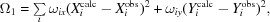where X and Y are the spot coordinates on the detector and ωix and ωiy are appropriate weights.

The parameters refined using spot positions are as follows.

 (i) Crystal-to-detector distance. (ii) Direct-beam position. (iii) A relative scale factor applied to the detector Y coordinates (YSCALE). (iv) Small rotations of the detector about a vertical and horizontal axis (TILT and TWIST). (v) Small rotation of the detector about the X-ray beam direction. (vi) Radial (ROFF) and tangential (TOFF) offsets for image-plate detectors with a spiral readout (e.g. MAR Research). (vii) Unit-cell parameters (optionally).
Note that it is not possible to refine changes in crystal orientation around the rotation axis using this residual, as this parameter has no effect on the spot positions. Other parameters, such as unit-cell parameters and crystal-to-detector distance, may also be highly correlated (depending on the maximum Bragg angle).

These parameters are normally refined independently for each image (or group of images) even though, with two possible exceptions, they might be expected to remain constant during data collection. The two parameters that could change are the crystal-to-detector distance (which can vary if the crystal is not centred exactly on the rotation axis, although this variation is unlikely to be more than 0.1 mm), and the direct-beam position (which can vary for image-plate detectors with multiple image plates, although the same plate should always have the same beam position). The YSCALE parameter would be expected to be exactly 1.0 for all modern detectors (where the pixel size is the same in the X and Y directions) and should be constant. The justification for refining these parameters is that this can compensate for errors in the unit-cell parameters. This is important when the initial unit-cell parameters obtained from autoindexing are being refined (see §4.2) and also when integrating the entire data set, as in both cases the accurate prediction of spot positions is crucial. There is now good evidence for a small but significant increase in unit-cell volume during data collection as the result of radiation damage (Murray & Garman, 2002; Ravelli et al., 2002). While in principle it is possible to refine the unit-cell parameters continuously to allow for this, in practice this is not reliable unless the symmetry is higher than orthorhombic. This is because for any given X-ray dose only data from a limited region of reciprocal space (a few images) are available to carry out the refinement and therefore not all unit-cell parameters will be well defined. Experience has shown that refinement of YSCALE and the crystal-to-detector distance compensates very well for genuine changes in unit-cell parameters and a smoothly changing decrease in the crystal-to-detector distance with increasing φ is a good indicator of a change in unit-cell parameters arising from radiation damage.

Some parameters (TILT, TWIST, ROFF, TOFF) are poorly defined for weak images (those with no strong spots in the outer regions of the detector) and can show large and random variations from one image to the next. In such cases, these parameters should be set to the average value and not refined during integration.

#### 4.2. Refinement using φ coordinates, post-refinement

In an ideal diffraction experiment with a strictly parallel monochromatic X-ray beam and a perfect crystal, the Ewald sphere is an infinitely thin spherical shell and the reciprocal-lattice points are infinitely small, so that each Bragg reflection occurs only at a precisely defined φ value (when the reciprocal-lattice point intersects the Ewald sphere). In reality, the divergence and wavelength dispersion of the X-ray beam result in an Ewald sphere with a finite thickness, while crystal mosaicity (and intrinsic variation in unit-cell parameters between different mosaic blocks) mean that each reciprocal-lattice point has a finite size. The combination of these effects means that each reflection has a finite reflecting range (or width) in φ. In most cases, the reflecting range is determined primarily by the mosaic spread of the crystal (particularly for cryocooled crystals that often have a mosaic spread greater than 0.3°) and by geometric factors that give rise to the Lorentz correction. This correction arises because for reciprocal-lattice points of any given size the φ rotation required for them to pass entirely through the Ewald sphere is proportional to their distance from the rotation axis. The practical result of this finite reflecting range is that reflections may be recorded only on a single image (fully recorded) or on two or more images (partially recorded) depending on the mosaic spread and the rotation angle per image (Fig. 4).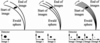Figure 4 Schematic representation of the effect of the finite size of reciprocal-lattice points on the diffraction images. The black ellipse represents a reciprocal-lattice point of finite size that results from the combination of crystal mosaicity, beam divergence, wavelength dispersion and variability in unit-cell parameters. The arcs represent the positions of the Ewald sphere at the beginning and end of one or more images. When the oscillation angle is large compared with the angular width of the reciprocal-lattice point (left), the entire reciprocal-lattice point lies between the two arcs and all the intensity is recorded on a single image (a fully recorded reflection). If the oscillation angle per image is halved (centre), the total intensity is distributed over two images (partially recorded reflections). If the oscillation angle is significantly less than the reflecting range, the intensity is distributed over several images (right), resulting in fine φ-slicing. Figure after Elspeth Garman.

Post-refinement (Winkler et al., 1979; Rossmann et al., 1979) uses the observed distribution of intensity of partially recorded reflections over adjacent images, together with a model of the rocking curve, to determine the exact φ value at which a given reciprocal-lattice point lies exactly on the Ewald sphere. It is called post-refinement because it can only be carried out after the images have been integrated. An absolute minimum of two images (adjacent in φ) is required to provide the necessary data.

The residual minimized in post-refinement is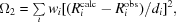where Ricalc and Riobs are the calculated and observed distances of the reciprocal-lattice point di* from the centre of the Ewald sphere (OP and OP′ in Fig. 5), respectively, and again wi is a weighting term. This represents the angle subtended by the points PP′ at the reciprocal-lattice origin (denoted δ in Fig. 5). Ricalc is determined from the current values for the unit-cell parameters and crystal orientation. Riobs is obtained from the φ centroid if fine φ-slices have been used. For coarse φ-slices, the position in φ of partially recorded reflections is estimated from the degree of partiality of the reflection [i.e. the way in which the total intensity is distributed between the two (or more) abutting images]. This latter approach requires a model for the rocking curve and permits refinement of either crystal mosaicity or beam divergence. Note that this residual can be used to refine crystal unit-cell parameters, orientation and mosaic spread, but cannot be used to refine the detector parameters.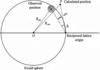Figure 5 The model for post-refinement in MOSFLM. The large circle represents a cross-section of the Ewald sphere. A reciprocal-lattice point is shown as a shaded circle centred on P′, representing its position at the end of the first of two images on which this reflection is recorded. The reciprocal-lattice point rotates clockwise during data collection, so the intensity recorded on the first image will be proportional to that part of the shaded circle that lies outside the Ewald sphere. P represents the calculated position of this reciprocal-lattice point using the current crystal parameters. The angle subtended by the points P, P′ at the origin of the reciprocal lattice is minimized during post-refinement of the crystal parameters.

Consider a reflection that spans two adjacent images. The position of the reciprocal-lattice point (which is rotating clockwise during data collection) is shown at the end of the first of the two images in Fig. 5. The reciprocal-lattice point can be modelled as a sphere with a radius given by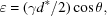where γ is the combined mosaic spread and beam divergence, d* is the reciprocal-lattice spacing and θ is the Bragg angle. The distance of the reciprocal-lattice point from the Ewald sphere, Δr, is related to the fraction P of the total intensity for this reflection that is recorded on the first image by a rocking curve model such as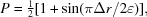where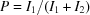and I1 and I2 are the intensities recorded on the two images. Knowing P from the measured intensities, Δr can be calculated and Robs can be determined. Rocking-curve models other than the simple sine function have also been used (e.g. Rossmann et al., 1979). Because depends on the combined mosaic spread and beam divergence, this parameter can also be refined. (For fine φ-slices the combined mosaic spread and beam divergence is estimated from the observed reflection width in φ.)

Typically, a few degrees of data in two segments widely separated in φ (ideally 90°) are required and refinement will provide unit-cell parameters that are accurate to within a few parts in 10 000 (for data that extend to at least 2.8 Å resolution). The crystal orientation will be correct to within a few hundredths of a degree for a mosaic spread less than 1.0° and is better determined for smaller values of the mosaic spread. For cubic symmetry a single segment of data is sufficient and this is also the case for trigonal, tetragonal or hexagonal crystals providing the unique axis lies close to the plane of the detector in the chosen segment. For monoclinic or triclinic crystals three (or four) segments of data (e.g. at Φ = 0, 45 and 90°) may be required to obtain accurate estimates of all unit-cell parameters.

In most cases post-refinement is not effective in refining the unit-cell parameters if the data only extend to low resolution (lower than 3.5 Å) and in such cases refinement using the spot positions will give more reliable results.

#### 4.3. Refinement strategy

The refinement strategy can depend on how the data have been collected. If fine φ-slices have been used, accurate φ centroids and coordinates (XY) are available for most strong reflections (excluding those very close to the rotation axis) and both residuals (Ω1, Ω2) can be minimized simultaneously using a suitable selection of reflections (strong and evenly distributed over the detector and in φ). Problems arising owing to correlations of different parameters can be avoided either by fixing some parameters or by the use of eigenvalue filtering. These problems can be particularly serious for low-resolution data, where there is a strong correlation between crystal-to-detector distance and the unit-cell parameters, or for an offset detector where there is a high correlation between the detector swing angle and the (horizontal) direct-beam coordinate. If only a narrow φ range of reflections is used in the refinement, then some unit-cell parameters will be poorly defined and may be correlated with the crystal setting angles and there will also be a strong correlation between the detector orientation around the X-ray beam and the crystal setting angle around the beam. In such circumstances the refined parameters may assume physically unrealistic values, but this will not necessarily reduce the accuracy of the prediction of reflection positions and widths.

When the data is collected with coarse φ-slices, accurate φ centroids can only be determined for partially recorded reflections. In MOSFLM the two residuals are currently minimized independently. Only the detector parameters are refined when minimizing the positional residual and only cell, orientation and mosaic spread parameters are refined by post-refinement. This approach does have the advantage that the accuracy of the refined unit-cell parameters does not depend on the accuracy of the crystal-to-detector distance or direct-beam position, providing these are known sufficiently well to allow correct indexing of the reflections. The unit-cell parameters are refined prior to integrating the images using two or more segments of data as described above and then fixed during integration. The effect of any inaccuracies in the refined cell, or changes in the cell owing to radiation damage, will be minimized by refinement of the crystal-to-detector distance and YSCALE.

### 5. Reflection integration

Once accurate values for the crystal unit-cell parameters and orientation have been obtained, the images can be integrated. Stated in the simplest way, this procedure involves predicting the position in the digitized image of each Bragg reflection present on that image and then estimating its intensity (after subtracting the X-ray background) and an error estimate of the intensity. In practice, this apparently simple task is quite complex.

#### 5.1. Predicting reflection positions

A knowledge of the crystal cell and orientation will allow the prediction of spot positions on a virtual detector; that is, a detector whose position and orientation are exactly known. These positions must then be mapped onto the digitized image and this mapping must take into account any spatial distortions introduced by the detector, either using a pre-determined calibration table or by refining the distortion parameters for each image. Accuracy in the prediction of spot positions is crucial, as any errors will introduce systematic errors in the integrated intensities, particularly for profile fitting. Ideally, unit-cell parameters should be known to an accuracy of better than 0.1%.

Typically, the detector parameters, crystal orientation and mosaic spread will be refined during the integration, but the unit-cell parameters will be fixed. The crystal orientation quite often changes during data collection, even with cryocooled crystals. This may be a consequence of the rapid acceleration/deceleration of the crystal at the start and end of each image, especially on high-intensity synchrotron beamlines with exposure times of less than 1 s. In addition, if the processing software assumes that the rotation axis is exactly orthogonal to the X-ray beam direction and this is not actually true, then the crystal orientation will appear to change smoothly with rotation angle with a period of 360°. Providing the changes in crystal orientation are gradual and are less than one-tenth of the mosaic spread between adjacent images, this will have no noticeable effect on data quality. Refining the mosaic spread during integration will allow for either anisotropy in the mosaic spread or an increase in mosaic spread arising from radiation damage.

#### 5.2. Two-dimensional and three-dimensional integration, coarse and fine φ-slicing

There are two distinct procedures for integrating spot intensities, known as two-dimensional and three-dimensional integration, that are available in different software packages; for example, MOSFLM (Leslie, 1992) and HKL (Otwinowski & Minor, 1997) use two-dimensional methods, while d*TREK (Pflugrath, 1999) and XDS (Kabsch, 1988) use the three-dimensional approach. These procedures differ in the way that the intensities of partially recorded reflections are derived. When using two-dimensional integration, the intensities of the different components of a partially recorded reflection (on different but adjacent images) are evaluated independently by two-dimensional profile fitting and only summed to give the total intensity when the data are merged and scaled. By contrast, when using three-dimensional integration the different components are assembled by the integration software and a three-dimensional profile is used to evaluate the total intensity.

Coarse and fine φ-slicing refer to different ways of collecting the images. Coarse φ-slicing describes the situation when the rotation angle per image is comparable to or greater than the mosaic spread (plus the beam divergence), so that the images will contain both fully recorded and partially recorded reflections. Fine φ-slicing corresponds to using a rotation angle per image that is significantly less than the mosaic spread, so that all reflections are partially recorded.

Data collected using fine φ-slicing can be processed using either two-dimensional or three-dimensional integration software. In principle, three-dimensional integration should give improved data quality, although in practice the difference between using two-dimensional and three-dimensional integration is marginal. Although three-dimensional integration programs are designed to process fine φ-sliced data, they can also be used to integrate coarse-sliced data effectively.

For weakly diffracting samples, a noticeable improvement in data quality can be obtained by collecting the images with moderately fine φ-slicing (rotation angle per image approximately one-third of the mosaic spread) compared with coarse φ-slicing. This is because the background included in each spot is minimized by using finer φ-slices, giving an improved signal to noise for weak reflections. However, the use of very fine φ-­slices is counterproductive, because of the intrinsic noise associated with each image (detector-readout noise) and because of systematic errors arising from difficulties in exactly synchronizing shutter movements with the spindle rotation. These issues have been discussed by Pflugrath (1999).

#### 5.3. Defining the peak/background mask

Because it is physically impossible to measure the X-ray background actually under the diffraction spot (which is strictly what is required to obtain the background-subtracted intensity), the background is measured in a region around the spot either in two dimensions (XY; the detector coordinates) for coarse φ-slices or in three dimensions (XY and φ) for fine φ-slices. A background plane is fitted to these background pixels and this plane is then used to estimate the background under the spot. To do this it is necessary to define a pixel mask which, when centred on the predicted position of the spot, will define which pixels are to be considered as part of the spot (the peak pixels) and which are to be used to determine the background (Fig. 6).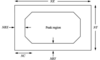Figure 6 The peak/background mask definition used by MOSFLM. The overall size of the mask (in pixels) is defined by NX and NY and the background region is defined by a background rim in X and Y (NRX and NRY pixels) and a corner cutoff (NC pixels).

The mask can be defined manually after visual inspection of the spot shapes, but MOSFLM will automatically optimize the peak/background definition. It is clearly important that pixels are not misclassified, as this can lead to systematic errors in the integrated intensity. The presence of strong diffuse scattering, which is quite commonly observed with data collected at a synchrotron, can lead to difficulties in differentiating between peak and background pixels. Unfortunately there is no simple way of dealing with this problem, although corrections can be applied when the data is scaled and merged (Evans, 2006).

#### 5.4. Summation integration and profile fitting

Having determined the background plane, the simplest way to obtain an estimate of the integrated intensity is to sum the pixel values of all pixels in the peak area of the mask and then subtract the sum of the background values for the same pixels calculated from the background plane. This is known as summation integration and if the background level is very low compared with the intensity of the spot and the spots are well resolved, this will give as accurate an estimate of the intensity as it is possible to obtain. (In such cases the accuracy is determined by counting statistics, so for a total count of N photons the standard deviation is N1/2.)

For weaker reflections, it is possible to obtain a more accurate estimate of the integrated intensity by using a procedure known as profile fitting (Diamond, 1969; Ford, 1974; Rossmann, 1979; Leslie, 1999). In this procedure, it is assumed that the shape or profile (in two or three dimensions) of the spots is known. The background plane is determined in the same way as for summation integration, but the intensity is derived by determining the scale factor which, when applied to the known spot profile, gives the best fit to the observed spot profile. This scale factor is then proportional to the profile-fitted intensity for the reflection. In practice, the fitting is performed by least-squares methods to minimize the residual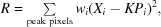where Xi is the background-subtracted intensity at pixel i, Pi is the value of the standard profile at the corresponding pixel, wi is a weight derived from the expected variance of Xi and K is the scale factor to be determined

The improvement obtained by profile fitting rather than summation integration depends on the spot intensity relative to background and the spot shape, but typically it can provide a reduction in variance by a factor of 2.0 (1.4 in the standard deviation) for weak reflections. It can be shown that for weak reflections the use of profile fitting effectively weights down the peripheral peak pixels where the signal to noise is lowest (Leslie, 1999). This can also be an advantage when adjacent spots are not completely resolved. All modern software packages employ profile fitting, although the implementation differs in detail.

The procedure assumes that the true reflection profile is known. In practice, this is determined from the observed reflection profiles of a number of reflections in the immediate vicinity of the reflection being integrated. An appropriate weighted sum of the individual profiles is used to form the true or standard profile. The reflection shape will vary with position on the detector (owing to changes in the obliquity of incidence and other factors) and it is important to allow for this. MOSFLM determines a standard profile for several predefined areas on the detector, using all reflections that lie on typically 5–10 adjacent images, to ensure that a significant number of reflections contribute to each profile. The inclusion of several images also means that the different components of partially recorded reflections will be included for the majority of the reflections. For the integration step, the best profile for each reflection is calculated as a weighted mean of the closest standard profiles. In HKL (Otwinowski & Minor, 1997), all spots that lie within a defined distance of the reflection being integrated on the current image are included in forming the profile. It should be noted that when using two-dimensional integration methods, it is not strictly correct to use a standard profile derived from fully recorded reflections to evaluate the intensity of partially recorded reflections, as the latter may have a different spot shape, but in practice this does not seem to have a noticeable effect on data quality. In the three-dimensional profile fitting employed by XDS, the profiles of individual spots are mapped back to a new coordinate frame based on the scattering vector for each spot (Kabsch, 1988). This elegant procedure eliminates the wide variation in the widths of different reflections in the φ direction, simplifying the tasks of both forming the standard profiles and fitting these to individual reflections. The same approach has been adopted in d*TREK (Pflugrath, 1999).

Profile fitting is a powerful technique for reducing the random error in weak diffraction data, but an error in determining the standard profiles or in fitting these to individual reflections will lead to systematic errors in all measured intensities (discussed in detail in Leslie, 1987). Modern software packages go to some lengths to minimize the magnitude of the systematic errors introduced by the use of non-ideal standard profiles. The most commonly observed problem affects the intensities of the strongest reflections when the spot size is very small (e.g. five pixels in width or smaller). In such cases the merging statistics can be better for the summation integration intensities than for the profile-fitted intensities. This is because the systematic errors arising from errors in the standard profile or in fitting this profile to an individual reflection are proportional to the reflection intensity (Leslie, 1987) and are the major determinant of the error in the profile-fitted intensity for strong reflections. In addition, the errors owing to artificial broadening of the standard profile or positional errors when fitting this profile to an individual reflection are proportionately greater for very small spots. As most integration programs output both the profile-fitted and summation integration estimates, the merging statistics can be compared and the appropriate estimate used when scaling and merging the data. Profile fitting will also introduce systematic errors if the spot shape is highly variable across the detector and this variation is smoothed out when forming the standard profiles. This can happen if the crystal is slightly split or if the crystal is physically bent as is sometimes the case for very thin crystals. In such cases it may be worthwhile to separately merge the summation integration and the profile-fitted estimates and compare the statistics for the downstream stages (phasing or refinement) using the two data sets.

#### 5.5. Standard deviation estimates

It is important to obtain reasonable estimates of the standard deviations of the integrated intensities, since these are used as weights when merging multiple observations and in subsequent steps of the structure determination (e.g. identification of heavy-atom sites, heavy-atom parameter refinement and model refinement). For summation integration and profile fitting of partially recorded reflections, a standard deviation can be obtained based on Poisson statistics, while for profile-fitted fully recorded reflections the goodness of fit of the scaled standard profile to the true reflection profile can be used (see Leslie, 1999, for a full derivation). In a total of N recorded X-ray photons, Poisson statistics states that the standard deviation is simply N1/2. To be able to apply Poisson statistics, it is necessary to convert the numbers in the digitized diffraction image to the equivalent number of X-ray photons (this conversion factor is referred to as the gain of the detector in documentation for the MOSFLM program). The gain is generally constant for a given make of detector and can be estimated by examining the variation in the counts within a small region of a diffraction image that does not include any diffraction spots, but corresponds only to the X-ray background. However, this estimate is only valid if all pixels are strictly independent of each other, an assumption that is not valid for commonly used image-plate and CCD detectors. In addition, variations in the sensitivity of different regions of the detector mean that it is an approximation to assume a single value for the gain. In spite of these difficulties, standard deviations based on Poisson statistics give reasonable estimates of the errors for weak and medium-intensity reflections, as judged by the agreement of the intensities of symmetry-related reflections. However, the situation is different for stronger reflections, where the difference between symmetry-related intensities is generally far greater than the estimated standard deviations. This is largely because the Poisson-based standard deviations only allow for random errors of measurement, while for strong reflections the systematic errors arising from absorption, beam instability, detector non-linearity or errors in non-uniformity corrections are far greater than the random errors. In MOSFLM, an additional contribution to the standard deviation is added to that based on Poisson statistics to try to model the systematic errors (Leslie, 1999). In spite of this, it is still generally necessary to modify the initial standard deviation estimates when the data are merged using the observed agreement between multiple observations (see Evans, 2006).

### Acknowledgements

The author wishes to thank Alan Wonacott, Peter Brick, Phil Evans, Jim Pflugrath and Harry Powell for many useful discussions relating to data processing. Work on the MOSFLM program is supported by CCP4.

### ReferencesBIOLOGICALCRYSTALLOGRAPHY